# srdanova math

#### msbiljanica

Joined Feb 14, 2012
23
questions
1.Z÷(10^n)=?,Z-integers
2.write in abbreviated form (if the function can be final and natural)
2+5=7 , 2+10=12 , 2+15=17, 2+20=22 , 2+25=27 , 2+30=32 , 2+35=37 , 2+38=40,
2+40=42, 2+41=43 , 2+44=46 , 2+45=47, 2+47=49 , 2+50=52 ,2+57=59 , 2+60=62 ,
2+64=66, 2+70=72, 2+71=73 , 2+78=80 , 2+80=82 , 2+85=87 , 2+90=92 ,2+92=94
3.how to solve this current knowledge of mathematics:
along a (20m) ,deleted between 10 m and 15 m (b=5m) , wet get c (image)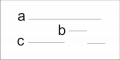Can mathematics explain the only two axiom that the rest are just evidence (experiments), if you think so join me show you Srdanova math, see you
________________________________________________________________
I figure this way, from education school has 12 years, I was always the subject of mathematics and physics had the best grades, math deal amateur, studying mathematics I came to know that mathematics can be simplified and be connected (to be explained only with two axiom) and extend the mathematics that can solve math problems that present no solution.Marjanovic Srdan
M.Biljanica
16201 Manojlovce
Serbia
<SNIP>
natural axiom
What is " nature along "?
-nature along in figure 1
What is "point"?
-start (end) natural long in figure 2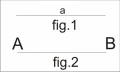What is the " basic rule "?
-basic rule is determined that the two ( more) longer only have to connect the points
[Sn]-mathematical facts
[S1]-nature along
[S2]-point (natural meaning of)
Definition[natural along]-two points , distance between two points
CM (current mathematics)-[S1]-does not know , [S2]-point is not defined , so anything and everything

Last edited by a moderator:

#### studiot

Joined Nov 9, 2007
4,998
Hello msbiljanica and welcome to All About Circuits.

I am not quite sure what points you are making here.

Could you separate them and post them one at a time?

#### msbiljanica

Joined Feb 14, 2012
23
Hello msbiljanica and welcome to All About Circuits.

I am not quite sure what points you are making here.

Could you separate them and post them one at a time?
Thank you for the welcome, this is like a like Euclid's Elements, in the simplest form, as geometric objects (the first natural geometrical object along the notion of point, the basic rule), all other evidence (slightly different than the current mathematics), which arise as a ratio of two (more) geometrical object formed from natural or along previous geometrical object. the point is that I think all of their math geometry, and that the numbers are a calculation function is actually another name for the related geometrical object
___________________
NATURAL MATHEMATICS

Presupposition-natural long merge points in the direction of the first natural along AB
Process:
P1-AB..CD..ABC(AC)
to read- natural along AB to point B, is connected to the natural long CD to point C, shall be
read- along the ABC(AC) to point C , connecting with the natural long DE to point D is done
renaming of points , we get along ABCD(AD)
...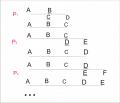[S3]-along (natural basis)
Definition[along]-the first and last point and the distance between points
CM-[S3]-does not know
__________________________________________________
Presupposition - All points of a longer (the infinite form) can be replaced with labels: (0), (0,1 ),...,
(0,1,2,3,4,5,6,7,8,9 ),...
Process:
P1-N (0) = {0,00,000,0000,...}
P2-N (0,1) = {0,1,10,11,100,...}
...
P10-N (0,1,2,3,4,5,6,7,8,9) = {0,1,2,3,4,5,6,7,8,9,10,11, ...}
...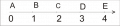[S4]-number along
[S5]-set of natural numbers N
We will use N (0,1,2,3,4,5,6,7,8,9) = {0,1,2,3,4,5,6,7,8,9,10,11,12,...}
Definition[number along]- a starting point (0), the last point at infinity
[number N]-The number 0 is the point 0
-Other numbers are longer, the first item is 0, the last point is the point of the name (number)
CM-[S4].does not know , [S5]-axiom
___________________________________
Presupposition-Numbers have their points
Process:
P1 0=(.0)
P2 1={(.0),(.1)}
P3 2={(.0),(.1),(.2)}
P4 3={(.0),(.1),(.2),(.3)}
P5 4={(.0),(.1),(.2),(.3),(.4)}
...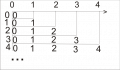[S6]-number points
CM-[S6]does not know

#### studiot

Joined Nov 9, 2007
4,998
In addition to the axioms of Euclid's Geometry, which were stated without proof there were a number of other supporting statements, also without proof.

In all there were

23 Definitions

5 Postulates

5 Common Notions or Axioms

There were also axioms added after Euclid, eg

"two straight lines do not enclose or contain a space."

Any new system will need to provide equivalents to all of these, which I suspect will add up to rather more than two statements made without proof.

You seem to be putting points on a line into one to one correspondence with the integers.
It is easy to show that there are point on a continuous line for which this cannot be done.

Last edited:

#### msbiljanica

Joined Feb 14, 2012
23
Presupposition-numbers have opposite points
Process:
P1 0=(s.0)
P2 1={(s.0),(s.1)}
P3 2={(s.0),(s.1),(s.2)}
P4 3={(s.0),(s.1),(s.2),(s.3)}
P5 4={(s.0),(s.1),(s.2),(s.3),(s.4)}
...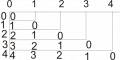...

[S7]-number opposite points
CM-[S7]does not know
_______________________________
Presupposition-numbers are comparable with each other
Process:
P1-two numbers (a, b ) are comparable with each other - a> b, a =b, a <b, ).(=(>,=,<)
P2-three numbers (a, b, c) are comparable with each other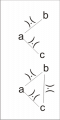P3-four numbers (a, b, c, d) are comparable with each other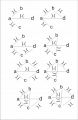...
[S8]-comparability numbers
CM-[S8]known two of comparability, comparability of three numbers(a number comparable with the numbers b and c),
comparability of the other knows.

#### studiot

Joined Nov 9, 2007
4,998
Please clear up something for me.

By "does not know" are you referring to what we call an open or closed set or interval?

An interval is part of a line or the set of all numbers in that part of a line.

An open interval does not contain its end points

A closed interval does

So for instance the open interval 0, 1 contains all the numbers between 0 and 1 but not 0 or 1 themselves.

So a closed interval contains all the numbers between 0 and 1 and 0 and 1 themselves.

We write the open inteval 0,1 as ] 0 , 1 [

and the closed interval 0,1 as [ 0 , 1 ]

to distinguish them.

Last edited:

#### msbiljanica

Joined Feb 14, 2012
23
"does not know"
calculation, or the name of a geometry object, and the rest, which does not exist in the current mathematics

#### studiot

Joined Nov 9, 2007
4,998

I am still not sure why you are trying to identify natural numbers with lines or is it points on a line?

As I pointed out in post#6 although all the numbers in N have equal significance, there are two types of points on a line and these types do not have equal significance.

#### msbiljanica

Joined Feb 14, 2012
23
I am still not sure why you are trying to identify natural numbers with lines or is it points on a line?
numbers are another name for the point (0) and longer (1,2,3,4, ....) of my math
____________________________

Presupposition-number ranges for number along
Process:
P1-image
P2-image
P3-image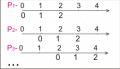[S9]-mobility of number
CM-[S9]-does not know
______________
Presupposition-Number (a) and mobile number (b ) but have no contact with the item
Process:
P1 ¤3(0)2¤
P2 ¤3(1)2¤
P3 ¤3(2)2¤
...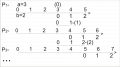Next - gap number and mobile number have no contact , except to point
...
[S10]-gap number GN={¤a(b)c¤,...,¤g(f)...(d)e¤}
[S11]-gap along
Definition[gap along] a>0-¤0(0)0¤-point
-¤0(a)0¤-two point ,separated by a gap
-¤a(0)0¤,¤0(0)a¤,¤a(0)a¤-along , two points
-¤a(a)a¤-two along , 4 points
...
CM-[S11],[S12] -does no know
________________
Presupposition-Number (a) and mobile number (b ) of a contat ,merge
Proces:
P1 3+(.0/.0)2=3
P2 3+(.1/.0)2=3
P3 3+(.2/.0)2=4 - image
P4 3+(.3/.0)2=5
form (.a/0) and (s.a/0) well continue this write (.a) and (s.a)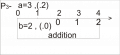P1 3+(.0)2=3
P2 3+(.1)2=3
P3 3+(.2)2=4
P4 3+(.3)2=5
General form
a+(.0)b=c
a+(.1)b=c
...
a+(.d)b=c
CM-only form a+(s.0/.0)b=c , others do not know , axiom

#### studiot

Joined Nov 9, 2007
4,998
What I understand you are saying is

Take a stick of length 4 units.
Place another stick of length 2 units on one end or altenatively
cut the first stick into two pieces and place the second stick between the pieces of the first.

Either way the result will be a length of 6 units?

Please correct this if I have missed something.

As regards the motivation for this,

You have used (assumed) several underlying properties from set theory about the natural numbers.

1)
If a and b are members of the set N then a+b = c is also a member of the set.
Furthermore c is unique. There is no other member, say d, for which a + b = d.

2)
N contains a member 0 such that a + 0 = a for every member of N
There is only one member 0 is unique.

3)
N contains a member (-a) such that a + (-a) = 0 for every member of N
Again (-a) is unique

#### msbiljanica

Joined Feb 14, 2012
23
Presupposition-Gap number is comparable with the gap number and number
Process:
P1 ¤a(b)c¤ , a+(s.0)c=z
P2 ¤a(b)c(d)e¤ , a+(s.0)c+(s.0)e=z
P3 ¤a(b)c(d)e(f)g¤ ,a+(s.0)c+(s.0)e+(s.0)g=z
...
number z as it compares as a number of
[S13]-comparability gap numbers
CM-[S13]-does no know
__________________________________________________
Presupposition-Adding the result can be written in short form:
a) a+(s.0)0 , a+(s.0)b , a+(s.0)b+(s.0)b , ...,a+(s.0)b+...+(s.0)b
b ) a+(s.0)b+...+(s.0)b,...,a+(s.0)b+(s.0)b, a+(s.0)b , a+(s.0)0
Process:
P1 - 3+(s.0)0=3 , 3+(s.0)4=7 , 347
3+(s.0)0=3 , 3+(s.0)4=7 , 3+(s.0)4+(s.0)4=11 , 3411
3+(s.0)0=3 , 3+(s.0)4=7 , 3+(s.0)4+(s.0)4=11 , 3+(s.0)4+(s.0)4+(s.0)4=15 , 3415
...
3+(s.0)0=3 , ... , 3+(s.0)4+...+(s.0)4=d , 34

P2 - 3+(s.0)4+(s.0)4+(s.0)4=15 , 3+(s.0)4+(s.0)4=11 , 3+(s.0)4=7 , 3+(s.0)0=3 , 1543
...
3+(s.0)4=7 , 3+(s.0)0=3 , 743
General form -abc , ab
[S14]-srcko
CM-[S14]-does no know
_______________________________________
Presupposition-Srcko can join a number not that can not be in the structure srcko
Process:
P1 101070 and 5 , 5_101070
P2 5520 and 22 ,5520_22
P3 75 and 25 , 75_25
P4 68 and 2 ,2_68
...
General form -abc_d , d_abc , ab_d ,d_ab...
[S15]-pendant srcko
CM-[S15]-does no know
Note-only one number can be pendand , number two goes into a complex srcko

#### msbiljanica

Joined Feb 14, 2012
23
Presupposition-Two ( more ) srcko (pendand srcko) are combined into one unit
Process:
P1 106 and 118 , 106118
P2 10565 and 703 ,10565_703
P3 30360 and 45277_78 ,30360_45277_78
...
General form -abcd , abc_de ,abc_def_g ,...
[S16]-two ( more) srcko
CM-[S16]-does no know
_______________________________
Presupposition-Two ( more ) srcko have the first ( last) common number
Process:
P1 10530 and 3330 , 10533(_30)
P2 4444 and 441094 and 44256 , 44(_44_)1094256
...
General form -abcd(_e) , ab(_c_)defg , ...
[S17]-two ( more) first-last srcko
CM-[S17]-does no know
______________________________________________________
Presupposition-In the expression a+(.b)c=d , d+(s.0)11 or d+(s.0)number (more) from 11
Process:
P1 3+(.s.0)5=8+(s.0)11 , 3+(s.0)5<91
P2 5+(.0)5=5+(s.0)224 , 5+(.0)5<729
...
General form - a+(.b)c=d+(s.0)11 ,a+(s.b)c<e1
a+(.b)c=d+(s.0)e , a+(.b)c<f
a+(.b)c=d+(s.0)efg , a+(.b)c<hij ...
[S18]-left inequality
CM-[S18]-know
_______________________________________
2+5=7 , 2+10=12 , 2+15=17, 2+20=22 , 2+25=27 , 2+30=32 , 2+35=37 , 2+38=40,
2+40=42, 2+41=43 , 2+44=46 , 2+45=47, 2+47=49 , 2+50=52 ,2+57=59 , 2+60=62 ,
2+64=66, 2+70=72, 2+71=73 , 2+78=80 , 2+80=82 , 2+85=87 , 2+90=92 ,2+92=94
srcko
5550={5,10,15,20,25,30,35,40,45,50}
38350={38,41,44,47,50}
501090={50,60,70,80,90}
50792={50,57,64,71,78,85,92}
two(more) first-last srcko
55383(_50_)1090792
remains part of the function, when we come to it

#### studiot

Joined Nov 9, 2007
4,998
I'm sorry but you have lost me somewhere along the way.

It would be very helpful if you could answer one my basic questions rather than ploughing on with more of your maths.

Otherwise I fear you are doing a lot of work for nothing.

mathematics can be simplified and be connected (to be explained only with two axiom) and extend the mathematics that can solve math problems that present no solution.
Please state what these two axioms are and how far they extend.

Please also state what problems conmventional maths cannot solve, but your system can, so that a comparison can be made.

That is pose a conventional maths problem in a conventional way.

Last edited:

#### msbiljanica

Joined Feb 14, 2012
23
Please state what these two axioms are and how far they extend.
You know the first axiom is called the natural along ,term point, the basic rule for linking the two (more) natural along.
second axiom when we come to it
all I ever show starts along the natural, or what became of natural along
2.write in abbreviated form (if the function can be final and natural)
2+5=7 , 2+10=12 , 2+15=17, 2+20=22 , 2+25=27 , 2+30=32 , 2+35=37 , 2+38=40,
2+40=42, 2+41=43 , 2+44=46 , 2+45=47, 2+47=49 , 2+50=52 ,2+57=59 , 2+60=62 ,
2+64=66, 2+70=72, 2+71=73 , 2+78=80 , 2+80=82 , 2+85=87 , 2+90=92 ,2+92=94

5550={5,10,15,20,25,30,35,40,45,50}
38350={38,41,44,47,50}
501090={50,60,70,80,90}
50792={50,57,64,71,78,85,92}
two(more) first-last srcko
55383(_50_)1090792
remains part of the function, when we come to it
a question for you if a function can be final and natural ?advantage of my mathematics

1.Z÷(10^n)=?,Z-integers
a={0,1,2,3,4,5,6,7,8,9} , b={1,2,3,4,5,6,7,8,9}
n=1 , Z÷10={...,(-2÷10),(-1÷10),(0÷10),(1÷10),(2÷10),...}={...,-0.2,-0.1,0,0.1,0.2,...}={Z,Z.b}
n=2 , Z÷100={Z,Z.b,Z.ab}
n=3 , Z÷1000={Z,Z.b,Zab,Zaab}
n=4 , Z÷10000={Z,Z.b,Z.ab,Zaab,Zaaab}
...
Z÷(10^n)=R
This evidence shows that the same rational and real numbers, that there are irrational numbers

#### msbiljanica

Joined Feb 14, 2012
23
Presupposition-Parts number (a) and mobile number (b ) have a contact , the contact is delete
Process:
P1 4-(.0)2=2
P2 4-(.1)2=¤1(2)1¤ image
P3 4-(.2)2=2
P4 4-(.3)2=¤3(1)1¤
P5 4-(.4)2=¤4(0)2¤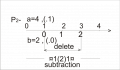General form
a-(.0)b=c
a-(.1)b=c
...
a-(.d)b=c
[S19]-subtraction
CM-only form a-(s.0/s.0)b=c , others do not know , axiom
_______________________________
3.how to solve this current knowledge of mathematics:
along a (20m) ,deleted between 10 m and 15 m (b=5m) , wet get c (image)20m-(.10m)5m=¤10m(5m)5m¤
How would you solve with current knowledge of mathematics

_________________--
Presupposition-In the expression a+(.b)c=d , d-(s.0/s.0))11f or d-(s.0/s.0))number (more) from 11f
Process:
P1 3+(.s.0)5=8-(s.0/s.0))118 , 3+(s.0)5>017
P2 5+(.0)5=5-(s.0/s.0))224 , 5+(.0)5>321
...
General form - a+(.b)c=d-(s.0/s.0))11f ,a+(s.b)c>01e
a+(.b)c=d-(s.0/s.0)e , a+(.b)c>f
a+(.b)c=d-(s.0/s.0)efg , a+(.b)c>hij ...
CM-[S20]-know
_________________________
Presupposition-Two ( more) addition (left and right inequalities) can be short to write
Process:
P1 3+(.013)4=y , 3+(.013)4>y ,3+(.013)4<y
P2 8+(.228)5=y , 8+(.228)5>y ,8+(.228)5<y
...
General form - a+(.bcd)e=y ,a+(.bcd)e>y , a+(.bcd)e<y
a+(.bcd_e)f=y , a+(.bcd_e)f>y , a+(.bcd_e)f<y ,...
CM-[S21]-does no know

#### studiot

Joined Nov 9, 2007
4,998
a question for you if a function can be final and natural ?advantage of my mathematics
1.Z÷(10^n)=?,Z-integers
Do I understand correctly by saying that I think you mean

Find an expression for Z such that Z is an integer or
Find all integers, Z, such that

$$\frac{Z}{{{{10}^n}}} \in {\rm N}$$

What do you mean by a function, a final function and a natural function?

#### msbiljanica

Joined Feb 14, 2012
23
Do I understand correctly by saying that I think you mean

Find an expression for Z such that Z is an integer or
Find all integers, Z, such that

$$\frac{Z}{{{{10}^n}}} \in {\rm N}$$

What do you mean by a function, a final function and a natural function?
$$\frac{Z}{{{{10}^n}}}= {\rm R}$$

#### studiot

Joined Nov 9, 2007
4,998
I'm sorry I don't understand your statement.

If you are asserting that the set of all integers, whether divided by some power of 10 or not, is isomorphic to the set of all real numbers

This is not the case.

There are more numbers in R than there are in Z.

#### msbiljanica

Joined Feb 14, 2012
23
Presupposition-Gap number ( value z) is a variable (same value) with the gaps that is constant
Process:
P1 ¤5(2)0¤,¤4(2)1¤,3(2)2¤,¤2(2)3¤,¤1(2)4¤,¤0(2)5¤ --5¤¤(2)

P2 ¤3(2)0¤,¤2(2)1¤,¤1(2)2¤,¤0(2)3¤
¤2(2)0¤,¤1(2)1¤.¤0(2)2¤
¤1(2)0¤,¤0(2)1¤
¤0(2)0¤ --013¤¤(2)

¤2(2)0¤,¤1(2)1¤,¤0(2)2¤
¤3(2)0¤,¤2(2)1¤,¤1(2)2¤,¤0(2)3¤
...
21¤¤(2)

[S22]-variability of z number
CM-[S22]-does no know
_______________
Presupposition-Translation of gap number in the variability z ( with constant gap ) can addition
(s.0)
Process:
P1 ¤3(2)3¤+(.z)¤4(2)4=6¤¤(2)+(.z)8¤¤(2)=14¤¤(2)
P2 ¤1(6)1(9)1¤+(.z)¤3(6)2(9)1¤=3¤¤(6)(9)+(.z)6¤¤(6)(9)=9¤¤(6)(9)
...
General form ¤a(b)c¤+(.z)¤d(b)e¤=f¤¤(b )+(.z)g¤¤(b )=h¤¤(b ) ...
CM-[S23]-does no know
__________________________________________
Presupposition-Translation of gap number in the variability z ( with constant gap ) can subtraction
(s.0/s.0)
Process:
P1 ¤3(2)3¤-(.z)¤1(2)1=6¤¤(2)-(.z)2¤¤(2)=4¤¤(2)
P2 ¤3(6)2(9)1¤-(.z)¤1(6)1(9)1¤=6¤¤(6)(9)-(.z)3¤¤(6)(9)=3¤¤(6)(9)
...
General form ¤a(b)c¤-(.z)¤d(b)e¤=f¤¤(b )-(.z)g¤¤(b )=h¤¤(b ) ...
[S24]-z subtraction
CM-[S24]-does no know

#### msbiljanica

Joined Feb 14, 2012
23
Presupposition-In the expression a-(.b)c=d , d+(.z)11¤¤e or d+(.z) number ( more ) from
11¤¤e , e={(f),(f)(f),(f)(f)(f),...}
Process:

P1 4-(.3)2=¤1(2)1¤+(.z)11¤¤(2) , 4-(.3)2<2¤¤(2)+(.z)11¤¤(2) , 4-(.3)2<31¤¤(2)
P2 4-(.3)2=¤1(2)1¤+(.z)7¤¤(2) , 4-(.3)2<2¤¤(2)+(.z)7¤¤(2), 4-(.3)<9¤¤(2)
...
General form
a#(.b)c=d+(.z)11¤¤e , a#(.b)c<s¤¤e+(.z)11¤¤e , a#(s.b)c<g1¤¤e
a#(.b)c=d+(.z)g¤¤e , a#(.b)c<s¤¤¤e+(.z)g¤¤e , a#(.b)c<l¤¤e
a#(.b)c=d+(.z)kpg¤¤e , a#(.b)c<s¤¤e+(.z)kpg¤¤e , a#(.b)c<hij¤¤e ..., #-calculation operations
(+,-,×,..)
[S25]-left inequality gap number
CM-[S25]-does no know
_____________________________________
Presupposition-In the expression a-(.b)c=d , d-(.z)11p¤¤e or d-(.z) number ( more ) from
11p¤¤e ,e={(f),(f)(f),(f)(f)(f),...}
Process:
P1 4-(.3)2=¤1(2)1¤-(.z)211¤¤(2) , 4-(.3)2>2¤¤(2)-(.z)211¤¤(2) , 4-(.3)2>011¤¤(2)
P2 4-(.3)2=¤1(2)1¤-(.z)1¤¤(2) , 4-(.3)2>2¤¤(2)-(.z)1¤¤(2) , 4-(.3)>1¤¤(2)
...
General form
a-(.b)c=d-(.z)11p¤¤e , a-(.b)c>s¤¤e-(.z)11p¤¤e , a-(s.b)c>g1k¤¤e
a-(.b)c=d-(.z)g¤¤e , a-(.b)c>s¤¤e-(.z)g¤¤e , a-(.b)c>l¤¤e
a-(.b)c=d-(.z)kpg¤¤e , a-(.b)c>s¤¤e-(.z)kpg¤¤e , a-(.b)c>hij¤¤e .. ,#-calculation operations
(+,-,×,...)
[S26]-right inequality gap number
CM-[S26]-does no know
_______________________________
Presupposition-The numbers are added , contact remains the rest is deleted
Process:

P1 4 - (.0)2=2
P2 4 - (.1)2=2 image
P3 4 - (.2)2=2
P4 4 - (.3)2=1
P5 4 - (.4)2=0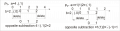P1 ¤1(1)2¤ - (.0)2=1
P2 ¤1(1)2¤ - (.1)2=1 image
P3 ¤1(1)2¤ - (.2)2=2
P4 ¤1(1)2¤ - (.3)2=1
P5 ¤1(1)2¤ - (.4)2=0
General form
a - (.0)b=c
a - (.1)b=c
...
a - (.d)b=c

[S27]-opposite subtraction
CM-[S27]-does no know